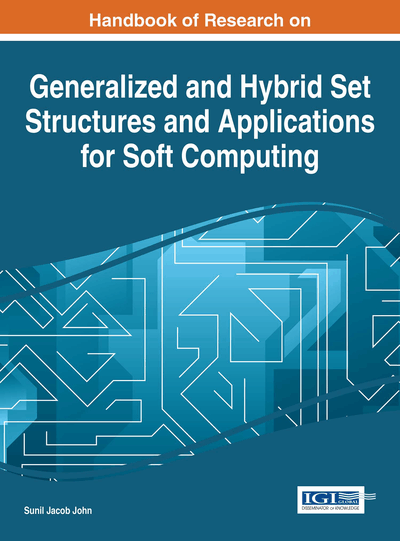# Soft Topologies Generated by Soft Set Relations

K. V. Babitha (College of Engineering Vadakara, India) and Sunil Jacob John (National Institute of Technology Calicut, India)
Copyright: © 2016 |Pages: 9
DOI: 10.4018/978-1-4666-9798-0.ch007
OnDemand PDF Download:
Available
\$37.50
No Current Special Offers

## Abstract

This chapter discusses different soft set topologies using binary soft set relations. The relationship between soft set topologies and different relations are investigated and some of the properties of these soft set topologies are obtained. The concept of topology generated by relations in the context of soft sets seems to be a promising tool for further research in the area of mathematics of soft sets.
Chapter Preview
Top

## 2. Preliminaries

In this section some basic definitions, results and notations as introduced by Molodtsov (1999), Maji et al. (2003), Irfan Ali et al. (2008), Babitha et al. (2010), Cagman (2011) are mentioned.

Definition 2.1 Let U be an initial universe set and E be a set of parameters. Let P (U) denotes the power set of U and AE. A pair (F, A) is called a soft set over U, where F is a mapping given by F: AP (U).

In other words, a soft set over U is a parameterized family of subsets of the universe U. For aA, F (a) may be considered as the set of a-approximate elements of the soft set (F, A).

Definition 2.2 Let (F, A) and (G, B) be two soft sets over U, then the Cartesian product of (F, A) and (G, B) is defined as (F, A) × (G, B) = (H, A × B), whereH:A × BP(U × U) and H(a, b) = F(a) × G(b), wherei.e.,Example 2.3 Consider the soft set (F, A) which describes the “cost of the houses” and the soft set (G, B) which describes the “attractiveness of the houses”.

## Key Terms in this Chapter

Soft Set Relations: Any subset of the Cartesian product of two soft sets.

Soft Sets: A structure introduced by Russian Mathematician Molodtsov in 1999 which makes an effective discretization of such fundamental mathematical concepts which are continuous in nature using parametrization techniques.

Soft Topology: Topology defined in the context of soft sets.

## Complete Chapter List

Search this Book:
Reset